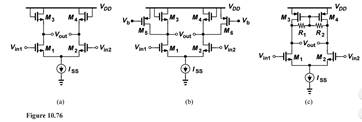### Create an Account

Home / Questions / Calculate the differential voltage gain of the circuits depicted in Fig 10 76 Assume perfe...

# Calculate the differential voltage gain of the circuits depicted in Fig 10 76 Assume perfect symmetry and

Calculate the differential voltage gain of the circuits depicted in Fig. 10.76. Assume perfect symmetry and λ > 0.Apr 24 2020 View more View LessSubscribe To Get Solution